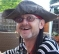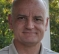## Deriving FIR coefficients for a higher sample rateStarted by 6 years ago17 replieslatest reply 6 years ago159 views

Hi folks

Does anyone have any tips on how I might transform a set of FIR coefficients from one sample rate to another at an integer multiple (2 in this case)?

Since the FIR coefficients are based on system measurements at a fixed sample rate, it is not possible to develop these from first principles.

I have been looking at simple direct interpolation of the coefficients (using sinc filtering etc) but I need to maintain the accuracy of the filtering action as well as possible, and I would like to be able to benefit from the aditional bandwidth which the higher sample rate offers; any interpolation filtering would seem to impair bandwidth and detail.

I have been investigating the possiblity of reverse engineering the coefficients into Magnitude and Group Delay, interpolating these, then using them to re-create a new FIR design at the new sample rate, but am having some difficulties with this; in particular deriving a suitable expression for phase from group delay which I can then use to design the FIR.

I can't help thinking that I have missed something very simple.

Many thanks for any suggestions or pointers.

Regards

Probbie

[ - ]Hi.  I wonder if the following "time-domain interpolation-by-M using frequency-domain zero stuffing" scheme will work for you [h(n) are your original FIR coefficients]:

• Perform an N-point DFT on an N-point h(n) time sequence, yielding N

frequency samples, H(m).

• Create an MN-point spectral sequence Hint(m) initially set to all zeros.

• Assign Hint(m) = H(m), for 0 ≤ m ≤ (N/2)–1.

• Assign both Hint(N/2) and Hint(MN–N/2) equal to H(N/2)/2. (This step,

to maintain conjugate symmetry and improve interpolation accuracy, is

not so well known.)

• Assign Hint(m) = H(q), where MN–(N/2)+1 ≤ m MN–1, and

(N/2)+1 ≤ q N–1.

• Compute the real part of the MN-point inverse DFT of Hint(m), yielding the desired MN-length interpolated hint(n) sequence.

• Finally, if desired, multiply hint(n) by M to compensate for the 1/M amplitude loss induced by interpolation.

[ - ]Hi Rick

That looks *really* interesting. I am currently working through it to see if I can use fft/ifft. Any pointers would be much appreciated.

Thanks so much, Probbie

[ - ]Hi Rick

That works for me! I didn't bother with trying to do it with fft/dft in the end since this is an offline operation. Just bought myself a copy of your book..

Have a beer on me.

Thanks so much, Probbie

[ - ]Hi probbie, I'm tickled the scheme I posted works for you. Thanks for the beer.  If you'd like I'll send you the errata for your copy of my book.  All you have to do is send me an e-mail at: R_dot_Lyons_@_ieee_org.

[ - ]I suggest using fft on your given filter then zero pad the bins either side to get twice frequency axis then back to time domain. Just a thought.

[ - ]Hi Kaz

Really appreciate the suggestion. That gives me alternate 'holes' in the magnitude response of the new FIR. I can't do simple linear interpolation on the complex response to fill the gaps of course.

Many thanks, Probbie

[ - ]Hi Probbie,

How about a simple interpolation of the coefficients?

Design a new FIR filter that is low pass with cutoff at quarter of the sampling rate.  Then divide this new impulse response into odd and even samples to create a polyphase pair of FIR filters.

Now convolve the FIR filter that you have through both sets, and reorder the results into one set that has twice as many coefficients, but the same frequency response as the desired at twice the sampling rate.  The only caution is getting the order right for the re-assembly.  Plot the result, and it should look nice and smooth.  If it looks jagged, then swap the order of the results.

Best of luck,

David

[ - ]Hi David,

%%%%%%%%%%%%%%%%%

h1 = fir1(20,.25);

h2 = conv(h1(1:2:end),h1(2:2:end));

freqz(h1);

freqz(h2);

%%%%%%%%%%%%%%%%%

h2 has wider band at normalised Fs

[ - ]Sorry, I seem to have caused confusion.

Use the two sets of coefficients to interpolate your existing FIR filter.

1) Your empirical FIR filter, that needs to be resampled, we will call H_orig

2) Design h1 as you have done with you MatLab code above (probably need more than 20 coeffs)

3) Select h2 = h1(1:2:end-1)

4) Select h3 = h1(2:2:end)

5) Convolve H_orig with h2

6) Convolve H_orig with h3

7) Reassemble H_origx2 by interleaving results from 5 and 6

David

[ - ]Hello David.  I've not heard of your scheme before.  It sounds very interesting and I'm gonna experiment using it. Thanks for posting your scheme!

[ - ]Hello Rick,

Thanks for your kind words.  Coming from someone who has contributed so many extremely useful ideas, I take your comment as a great compliment.

I am surprised by your response though, because I did not think my idea was non-obvious.

If the data we wanted to interpolate (by two in this case), were a signal, wouldn't we design an interpolation filter at the desired sampling rate, split it into two polyphase components, and then simply alternate between the two filters to create two outputs (simple sum-of-products) for every shift of the delay line?

Since we have the entire "signal" - in this case a set of FIR coefficients - we can do the entire convolution of each of the phases and then recombine.  Didn't seem like a big stretch to me.

I have spent many years working on various sample rate conversion schemes, including nasty ratios like 44.1 ksps to/from 48.0 ksps (147/160), and so I've had to figure out a few tricks along the way.

Thanks again.

David

[ - ]Hi David, thanks for the kind words.  The notion of using a polyphase FIR interpolation process never occurred to me! There see, ...that's why they pay you the big bucks and I only make thirty seven cents an hour.

[ - ]Thanks David,

It works. Interesting method but hard for me to understand its principles.

[ - ]That was quick.

The principle is simple.

Normally to up-sample, we would add zeros between existing samples, and run through the newly designed h1.

Instead we keep the spacing of the existing FIR H_orig, and create two different phases (hence polyphase) of the new oversampling filter.  (This process avoids any multiplies by the inserted zeros.)  We convolve to create two different phases of the interpolated H_orig filter.  Now we just have to reassemble the two phases into one longer oversampled filter.

Voila!

For higher up-sampling rates, we just need to reduce the cutoff of the h1 design.

Great!

David

[ - ]Hi David

Really appreciate the suggestion. I believe what you are suggesting is a more efficient implementation of interpolation. Efficiency isn't a big requirement for me since this is an 'offline' operation. I have tried various forms of interpolation but I haven't yet found a form which preserves the filtering action or bandwidth sufficiently well.

Many thanks, Probbie

[ - ]If your filter has n taps and you use fft method I suggest:

you use n fft resolution (you may use high resolution but then it requires truncation).

Pad the fft bins on either side of dc with last bin value (instead of zero) then do 2*n ifft and pick up the 2*n coefficients from amplitude of ifft output then scale for unity (dc gain of 2)

[ - ]